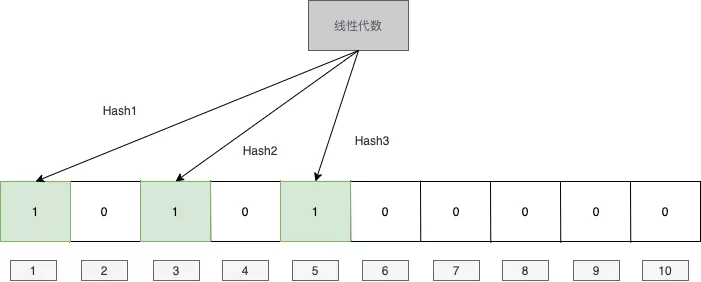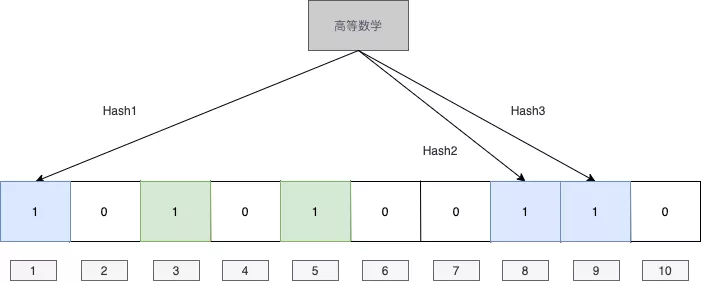# 大数据算法——布隆过滤器

2019/04/10 10:10

<br>

<br>

## 原理

<br><br>

## 代码

<br>

# 插入元素
def BloomFilter(filter, value, hash_functions):
m = len(filter)
for func in hash_functions:
idx = func(value) % m
filter[idx] = True
return filter

# 判断元素
def MemberInFilter(filter, value, hash_functions):
m = len(filter)
for func in hash_functions:
idx = func(value) % m
if not filter[idx]:
return False
return True


<br>

## 错误率计算

<br>

$\displaystyle\left[1-(1-\frac{1}{m})^{nk}\right]^k \approx (1-e^{-\frac{kn}{m}})^k$

$\displaystyle\lim_{x \to -\infty}(1-\frac{1}{x})^{-x}=e$

$f(k) = (1-b^{-k})^k \ \ln f(k) = k\ln(1-b^{-k})$ 两边求导：

\begin{aligned} \frac{1}{f(k)}f'(k)&= ln(1-b^{-k}) + \frac{kb^{-k}\ln b}{1-b^{-k}} \end{aligned}

\begin{aligned} \ln(1-b^{-k})(1-b^{-k})&=-kb^{-k}\ln b\ \ln(1-b^{-k})(1-b^{-k})&=b^{-k}\ln b^{-k}\ 1-b^{-k} &=b^{-k}\ b^{-k} &= \frac{1}{2} \end{aligned}

<br>

## 灵魂拷问

<br>### 作者的其它热门文章

0
0 收藏

0 评论
0 收藏
0